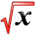# Integration TechniquesThis article is part of the MathHelp Tutoring Wiki

1. To demonstrate the different integration techniques
2. To explain when to apply each of the techniques

## Substitution

Two rules of thumb for the substitution techniques:

1. treat this as a default technique, ie, always try substitution first
2. let u = "the most complicated term". For example:
• Q: Integrate ∫ (6$x^{2}$+ 6x - 5)/(2$x^{3}$+ 3$x^{2}$-5x - 1) dx.
• A: The easy way is to let u = 2$x^{3}$+ 3$x^{2}$-5x - 1 and try it out (the hard way is to use partial fraction and try to factorize the denominator). We have du = (6$x^{2}$+ 6x - 5) dx, and sub it in to get answer ln |(2x3 + 3$x^{2}$-5x - 1)| + C

However, there are exceptions to the rules of thumb:

• Q: Integrate ∫ x/(1 + $x^{4}$) dx.
• A: First substitute u = x2. We know du = 2x dx, so the integral becomes ∫ 1/2(1 + u2). Now use the arctan formula to get 1/2 arctan u. The answer is 1/2 arctan (x2) + C.

## Integration by Parts

Integration by parts come from product rule of differentiation. Recall production rule of differentiation of two functions (uv)' = u'v + uv'. We can rearrange the terms to u'v = (uv)' - uv' and integrate both sides. We get ∫ u'v = uv - ∫ uv'.

• Q: Ingegrate x*$e^{x}$*dx
• A:

First break it into two parts, one part is x*dx, the other part is $e^{x}$.

so du=$e^{x}$*dx, v=x

then, we can calculate u=$e^{x}$, v=dx

so ∫ u'v=uv-∫ uv'

=xex-∫ $e^{x}$dx

=x$e^{x}$-$e^{x}$=(x-1)*$e^{x}$+ C

## Partial Fraction

Partial fraction method is used when we are integrating rational functions, ie, a polynomial divided by a polynomial.

However, note that partial fraction method only works well when the degree in the numerator is smaller than the degree in the denominator. When we get the other case (degree numerator > degree denominator), we have to do long division. For example:

• Q: ∫ f(x) dx, where f(x) = (2$x^{3}$- 2$x^{2}$+ 9x -1)/($x^{2}$- x - 2)
• A: As the numerator is of degree 3, which is larger than the degree-2 denominator, we have to do long division to get f(x) = 2x + (5x-1)/(x2 - x - 2). Then we want to break down the rational function (5x-1)/($x^{2}$- x - 2) = (5x - 1)/(x-2)(x+1) = A/(x-2) + B(x+1). Solving the equation by substituting x = 2 and x = -1 to get (5x-1)/($x^{2}$- x - 2) = 3/(x-2) + 2/(x+1). So the integral is ∫ 2x + 3/(x-2) + 2/(x+1) dx = $x^{2}$+ 3ln|x - 2| + 2ln|x+1| + C

## Trigonometric Substitution

Trigonomitric substitution is used when we have trigonometric functions or the problem takes the form $x^{2}$+ $a^{2}$, $x^{2}$- $a^{2}$, or $a^{2}$- $x^{2}$.

(try variations like completing square)

And there are two general types of trig integrals

A. calculate ∫ (sinx)m(cosx)ndx where m,n are both positive integers

A1. n is odd, m doesn't matter.

• Q: ∫ (sinx)2(cosx)3dx
• A:we can isolate one cos(x) from the function, and then use integration by parts.

∫ (sinx)2*(cosx)3dx

=∫ (sinx)2(cosx)2cosx dx

use (sinx)2+(cosx)2=1

so that (cosx)2=1- $(sinx)^{2}$if u=sinx, du= cosx*dx

then the original function will be

=∫ $u^{2}$*(1-$u^{2}$)*du

Notes: The strategy is to isolate cosx, and use (cosx)2=1- (sinx)2

Denote u=sin(x), du=cos(x)*dx

so the function will become ∫ polynomial(u)*du

A2. m is odd, n doesn't matter

• Q: ∫ (sinx)5(cosx)3dx
• A: we can isolate sinx from the function and then use integration by parts.

=∫ (sinx)4(cosx)3sinxdx

Denote u=cosx, du=sinxdx

=-∫ (sinx)4*(cosx)3dx

=-∫ (1-$u^{2}$)2*$u^{3}$*du

since sinx2=1-cosx2=1-u2

Notes: The strategy is to isolate sinx, and use (sinx)2=1-(cosx)2

Denote u=cosx, du=-sin(x)dx

A3. Both m and n are even numbers

Strategy: Use (cosx)2=(cosx+1)/2, (sinx)2=(1-cosx)/2

• Q:

∫ (sinx)2*(cosx)2dx

• A:

=∫ (cos2x+1)(sin2x+1)dx

=1/4 ∫ (1-cos2x)2dx

=(1/4)x- 1/4 ∫ cos2x*dx

=(1/4)x-1/4 ∫ (1+cos4x)/2*dx

=(1/4)x-1/2x-1/8 ∫ cos4x*dx

=-(1/4)x-(1/8)(sin4x)*(1/4) +C

## Challenging problems

• Q: Solve the following problem by both substitution and integration by parts: ∫ sin(sqrt(x)) dx.
• A: First we make a substitution u = sqrt(x), ie, du = 1/[2sqrt(x)] dx = dx/2u. Substitute this in to get int 2u*sin (u) du. Integration by part gives -2ucos(u) + int cos(u) du = -2u cos u + sin(u)+ C. Sub u = sqrt(x) back to get the answer.